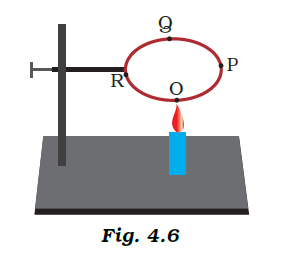# A circular metal loop is heated at point O as shown in Figure (i) In which direction would heat flow in the loop? (ii) In which order the pins at points P,Q and R fixed with the help of wax fall if points O, P, Q and R are equidistant from each other?(i) Heat flows in solids at the same rate in all directions, hence heat will flow from O to P and likewise from O to R.
(ii)The heat first travels to R and P, causing the pins R and P to fall first. After that, the heat travels to point Q, causing the pin Q to fall.(0)(0)